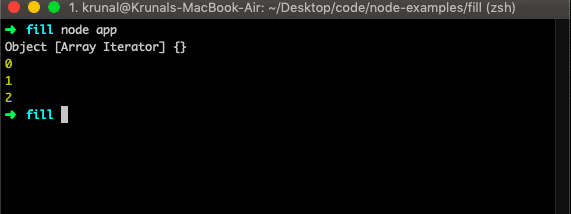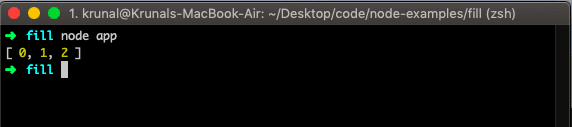# How to Get Keys of an Array in JavaScript

JavaScript array keys() method returns an Array Iterator object with the keys of an array.

## JavaScript Array keys

To get the keys of an array in JavaScript, use the array.keys() method. Javascript array keys() method returns the new Array Iterator object that contains the keys for each index in an array. The array keys() method does not change the original array.

### Syntax

The syntax for the Javascript Array keys() method is the following.

`arr.keys()`

Let us take a simple example.

```// app.js

let arr = ['a', 'b', 'c'];
let iteratorObj = arr.keys();

console.log(iteratorObj);```

So, it has given us iteratorObj. We can get the values by iterating for..of() loop.

```// app.js

let arr = ['a', 'b', 'c'];
let iterator = arr.keys();

console.log(iterator);

for (let key of iterator) {
console.log(key);
}
```We can get the array of keys by the following code.

```// app.js

let arr = ['a', 'b', 'c'];
let iterator = arr.keys();
let keyArray = [];

for (let key of iterator) {
keyArray.push(key);
}
console.log(keyArray);
```To find the keys of the Object, use the Object.keys() function in JavaScript.

## Length of a Javascript associative array

In JavaScript, there are two types of arrays: Standard arrays and The associative arrays

1. [ ] – standard array – 0-based integer indexes only.
2. { } – associative array – javascript objects where keys can be any strings.

You can define a standard array where indexes can only be integers.

When you do arr[“something”], since something (which is what you use as index) is not an integer, you are defining a property to the arr object (everything is an object in javascript). But you are not adding an element to the standard array.

You can use the array notation to refer to object properties, which the solutions in this thread show. What is created instead is an iterable object.

The elements in an associative array are ordered in their declared order. With iterable objects, browsers each have their method of sorting the items in an iterable object.

We can not find the length of an associative array or object directly.

So we need to use the Object.keys() function and then use the length property to find the length.

See the following code.

```let shows = new Array();

shows["s1"] = "StrangerThings 1";
shows["s2"] = "StrangerThings 2";
shows["s3"] = "StrangerThings 3";

console.log(Object.keys(shows).length);```

That’s it.

### 1 thought on “How to Get Keys of an Array in JavaScript”

1.thanks for the post